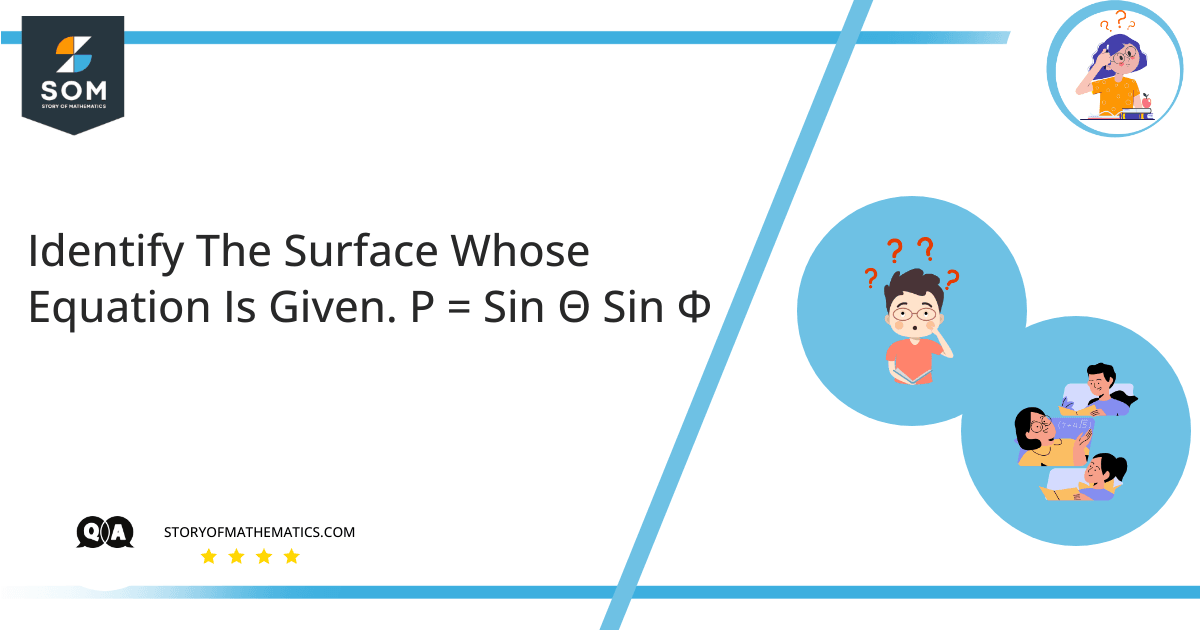# Identify the surface whose equation is given. ρ=sinθsinØThe aim of this question is to find the surface corresponding to the Spherical Coordinates $p=sin\theta sin\phi$ by utilizing the Cartesian Coordinate system and Equation of Sphere.

First, we will explain the concept of Sphere, its Equation, and its Coordinates in the Cartesian Coordinate System.

A Sphere is defined as a $3D$ geometrical structure have a constant radius $\rho$ across all three dimensions and its center point is fixed. Therefore, the equation of sphere is being derived by considering the position coordinates of sphere centers with their constant radius $\rho$

${(x-a)}^2+{(y-b)}^2+{(z-c)}^2= \rho^2$

This is the Equation of Sphere where

$Center = A(a, b, c)$

$Radius = \rho$

For a Standard Sphere in standard form, we know that the center has coordinates as $O(0,0,0)$ with $P(x,y,z)$ being any point on the sphere.

$A(a, b, c) = O(0, 0, 0)$

By substituting the coordinates of the center in the above equation we get:

${(x-0)}^2+{(y-0)}^2+{(z-0)}^2= \rho^2$

$x^2+y^2+z^2= \rho^2$

In Cartesian Coordinate System, we convert the equation given in spherical coordinates to rectangular coordinates to identify its surface.

In physics, $\theta$ is defined as the Polar Angle (from the positive z-axis) and $\phi$ is defined as the Azimuthal Angle. By utilizing the concept of spherical coordinates, we know that a sphere having a radius  is defined by 3 coordinates

$x=\rho\ sin\theta\ cos\phi$

$y=\rho\ sin\theta\ sin\phi$

$z=\rho\ cos\theta$

Given As:

$p= sin\theta\ sin\phi$

By multiplying both sides with $\rho$, we get

$\rho^2= \rho\ sin\theta\ sin\phi$

As we know as per the Cartesian Coordinate System

$y= \rho\ sin\theta\ sin\phi$

Hence,

$\rho^2=y$

By substituting the value of $\rho^2$ in the Equation of Sphere, we get:

$x^2+y^2+z^2 = y$

$x^2+y^2-y+z^2 = 0$

Adding $\dfrac{1}{4}$ on both sides:

$x^2+{(y}^2-y+\dfrac{1}{4})+z^2 = \dfrac{1}{4}$

As we know that:

$y^2-y+\dfrac{1}{4} = {(y-\dfrac{1}{2})}^2$

By substituting the value in above equation

${(x-0)}^2+{(y-\dfrac{1}{2})}^2+{(z-0)}^2 = {(\dfrac{1}{2})}^2$

By comparing it with the equation of sphere

${(x-a)}^2+{(y-b)}^2+{(z-c)}^2 = \rho^2$

We get the coordinates for the center of the sphere and radius $\rho$ as follows:

$Center\ A(a, b, c)=A(0, \dfrac{1}{2}, 0)$

$Radius\ \rho= \dfrac{1}{2}$

## Numerical Result

The surface that corresponds to $p=sin\theta sin\phi$ is a Sphere with $Center\ A(a, b, c)=A(0, \dfrac{1}{2}, 0)$ and $Radius\ \rho=\dfrac{1}{2}$.Figure 1

## Example

Identify the surface whose equation is given as $r = 2sin\theta$

We know that:

Cylindrical Coordinates $(r,\theta,z)$ with Center $A(a, b)$ are represented by equation:

${(x-a)}^2+{(y-b)}^2 = r^2$

$\tan{\theta = \dfrac{y}{x}}$

$z=z$

Where:

$x= rcos\theta$

$y= rsin\theta$

Given that:

$r= 2sin\theta$

$r^2=4\sin^2\theta$

$r^2=2sin\theta\times2sin\theta=2sin\theta\times \ r=2rsin\theta$

Substituting the value of $y=rsin\theta$, we get

$r^2=2y$

Putting the value in the equation of Cylindrical Coordinates, we get

$x^2+y^2=2y$

$x^2+y^2-2y=0$

Adding $1$ on both sides

$x^2+(y^2-2y+1)=1$

$x^2+(y^2-2y+1)=1$

As we know that:

$y^2-2y+1={(y-1)}^2$

By substituting the value in the above equation

${(x-0)}^2+{(y-1)}^2=1$

We get the coordinates for the center of the circle and radius $r$ as follows:

$Center\ A(a,b)=A(0,1)$

$Radius\ r=1$

Hence, the surface that corresponds to $r=2sin\theta$ is a circle with $Center\ A(a,b)=A(0,1)$ and $Radius\ r=1$.Figure 2

Image/Mathematical drawings are created in Geogebra.